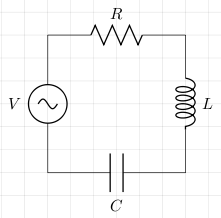# Resonance

The following is a LCR series circuit (L stands for inductor, C for capacitor, and R for resistor).The inductive reactance is

```	```
XL = ωL
```
```

The capacitive reactance is

```	```
XC = 1/(ωC)
```
```

The impedance is

```	```
Z = R +j(XL-XC)
```
```

The impedance is at a minimum when `XL=XC` which happens when

```	```
ωL = 1/(ωC)
ω2L = 1/C
ω2 = 1/(LC)
ω = 1/√(LC)
```
```

This is known as the resonant frequency. At this point the instantaneous current is at its maximum and in phase with the instantaneous voltage.

The bandwidth — the range of frequencies either side of `ω` where the instantaneous current is above the cut-off frequency — is

```	```
Δω = R/L
```
```

The Q factor gives us an indication of the sharpness of the current peak. A high Q factor is a sharp peak, and a low Q factor is a broad peak. This can be calculated as follows:

```	```
Q = ωL/R
= 1/(RωC)     [ωL = 1/(ωC)]
= 1/R×√(L/C)
```
```

The selectivity of the circuit is given by the Q factor. Also, at the resonant frequency:

```	```
VC = VL = QV
```
```

## References

Fischer-Cripps. A.C., The Electronics Companion. Institute of Physics, 2005.